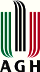# List of talks

## Plenary lectures

Karol Baron, Linear iterative functional equations and sequences built of iterates

Zuzana Došlá, Boundary value problems to functional difference equations on unbounded domains

Tomasz Downarowicz, Multiplicative normality

Michał Misiurewicz, Variations on the Sharkovsky Theorem

## Contributed talks

Francisco Balibrea, ﻿On homoclinic and heteroclinic chaos on plane discrete systems

Jan Boroński, Recent advances on minimal systems and minimal spaces

Henk Bruin, Regular variation for almost Anosov diffeomorphisms

Janusz Brzdęk, Ulam stability and fixed point theorems

Carlo Carminati, Matching for generalised β-transformations

Jacek Chmieliński, Birkhoff orthogonality and its reversal

Jacek Chudziak, On a derivation of the Goldstein-Einhorn probability weighting functions

Welington Cordeiro, Generalizations of expansivity

Jimmy Devillet, On biselective operations

Bartosz Frej, Doubly stochastic operators with zero entropy

Roman Ger, Translation equation viewed as the Cauchy one

Dorota Głazowska, Invariance of quasi-arithmetic mean with respect to weighted Bajraktarević mean-type mappings

Chris Good, The preservation of shadowing by factor maps

Karol Gryszka, On certain generalization of asymptotic periodicity

Eszter Gselmann, Characterization of field homomorphisms through Pexiderized functional equations

Pierre-Antoine Guihéneuf, Discretizations of expanding maps. A dynamical approach of Hilbert's 6th problem

Jonathan Hoseana, The mean-median map

Eliza Jabłońska, On Steinhaus properties in Abelian Polish groups

Wojciech Jabłoński, Groups of commuting formal power series and the third Aczél-Jabotinsky differential equation

Justyna Jarczyk, Joining means by iteration

Witold Jarczyk, Embeddability of pairs of weighted quasi-arithmetic means in a semiflow

Olena Karpel, Exact number of ergodic invariant measures for Bratteli diagrams

Christoph Kawan, State estimation and entropy of nonautonomous systems

Gergely Kiss, Functional equations that characterize higher order derivations

Derong Kong, Bifurcation sets arising from non-integer base expansions

Agnieszka Malinowska, The Hegselmann-Krause opinion formation model with predictive mechanisms

Joel Mitchell, The Auslander-Yorke Dichotomy revisited

Janusz Morawiec, Attractor of Cantor type with positive measure

Issam Naghmouchi, Dynamics of regular curves homeomorphisms

Kazuki Okamura, Some results for conjugate equations

Andrzej Olbryś, On the characterization of convex differences

Piotr Oprocha, Dynamical properties of maps on quasi-graphs

Zsolt Páles, On the nontrivial solutions of countable functional equation systems

Paweł Potorski, Mixing properties in expanding Lorenz maps

Barbara Przebieracz, Approximate homomorphisms on lattices

Peter Raith, Stability of pressure and entropy for monotonic mod one transformations

Maciej Sablik, Non-symmetric equations stemming from MVP

Cristina Serpa, Fractal structure of iterative functional equations

Puneet Sharma, On dynamics of non-autonomous discrete dynamical systems

Yong-Guo Shi, Introduction to one of Zdun's contributions: on conjugacy of some systems of function

Artur Siemaszko, Coexistence of orbits of different topological types in topologically transitive cylinder transformations

Ľubomír Snoha, ﻿Dynamical consequences of a free interval

Marta Štefánková, On generic properties of nonautonomous dynamical systems

Mariusz Sudzik, An equation with rescaling and its solutions attaining an absolute extreme

Tomasz Szostok, On Levinson's inequality

Imke Toborg, On the functional f(x)+f(y)=max{f(xy),f(xy-1)} equation on groups

Marek Cezary Zdun, On singular iteration groups

Yingying Zeng, The oscillation of smooth iterative roots